Topics: AI | Data Science | Data Visualization | Deep Learning | Machine Learning | NLP | Python | R | Statistics

KDnuggets Home » News » 2018 » Nov » Tutorials, Overviews » Multi-Class Text Classification Model Comparison and Selection ( 18:n42 )

# Multi-Class Text Classification Model Comparison and Selection

This is what we are going to do today: use everything that we have presented about text classification in the previous articles (and more) and comparing between the text classification models we trained in order to choose the most accurate one for our problem.comments

### Word2vec and Logistic Regression

Word2vec, like doc2vec, belongs to the text preprocessing phase. Specifically, to the part that transforms a text into a row of numbers. Word2vec is a type of mapping that allows words with similar meaning to have similar vector representation.

The idea behind Word2vec is rather simple: we want to use the surrounding words to represent the target words with a Neural Network whose hidden layer encodes the word representation.

First we load a word2vec model. It has been pre-trained by Google on a 100 billion word Google News corpus.

```from gensim.models import Word2Vec

wv.init_sims(replace=True)```

We may want to explore some vocabularies.

```from itertools import islice
list(islice(wv.vocab, 13030, 13050))```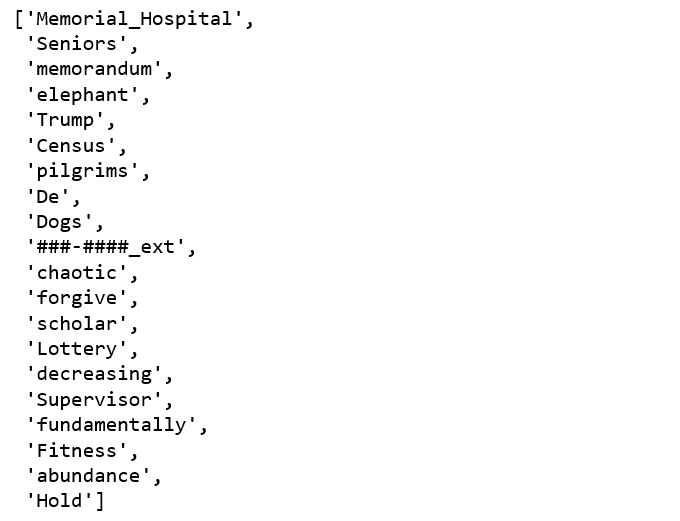Figure 9

BOW based approaches that includes averaging, summation, weighted addition. The common way is to average the two word vectors. Therefore, we will follow the most common way.

We will tokenize the text and apply the tokenization to “post” column, and apply word vector averaging to tokenized text.

Its time to see how logistic regression classifiers performs on these word-averaging document features.

```from sklearn.linear_model import LogisticRegression
logreg = LogisticRegression(n_jobs=1, C=1e5)
logreg = logreg.fit(X_train_word_average, train['tags'])
y_pred = logreg.predict(X_test_word_average)
print('accuracy %s' % accuracy_score(y_pred, test.tags))
print(classification_report(test.tags, y_pred,target_names=my_tags))```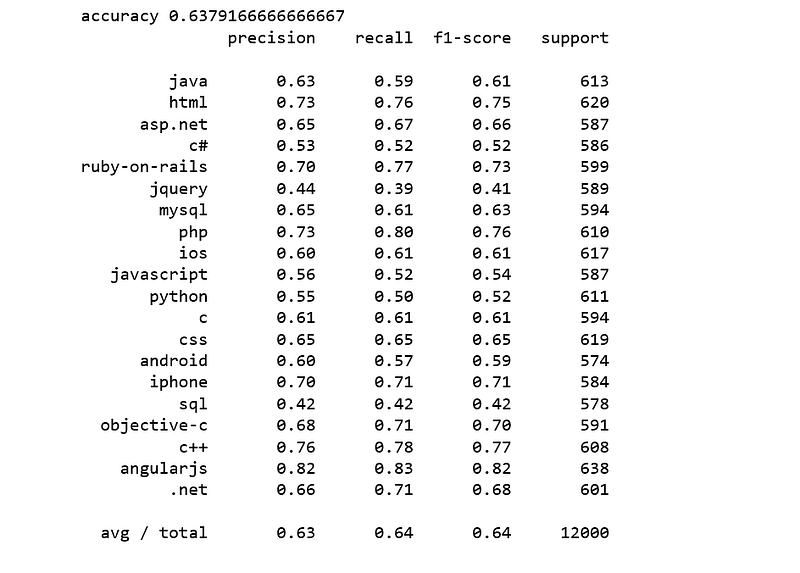Figure 10

It was disappointing, worst we have seen so far.

### Doc2vec and Logistic Regression

The same idea of word2vec can be extended to documents where instead of learning feature representations for words, we learn it for sentences or documents. To get a general idea of a word2vec, think of it as a mathematical average of the word vector representations of all the words in the document. Doc2Vec extends the idea of word2vec, however words can only capture so much, there are times when we need relationships between documents and not just words.

The way to train doc2vec model for our Stack Overflow questions and tags data is very similar with when we train Multi-Class Text Classification with Doc2vec and Logistic Regression.

First, we label the sentences. Gensim’s Doc2Vec implementation requires each document/paragraph to have a label associated with it. and we do this by using the `TaggedDocument` method. The format will be “TRAIN_i” or “TEST_i” where “i” is a dummy index of the post.

According to Gensim doc2vec tutorial, its doc2vec class was trained on the entire data, and we will do the same. Let’s have a look what the tagged document looks like:

`all_data[:2]`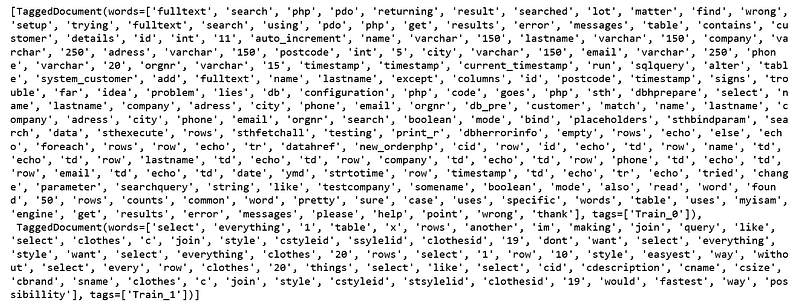Figure 11

When training the doc2vec, we will vary the following parameters:

• `dm=0` , distributed bag of words (DBOW) is used.
• `vector_size=300` , 300 vector dimensional feature vectors.
• `negative=5` , specifies how many “noise words” should be drawn.
• `min_count=1`, ignores all words with total frequency lower than this.
• `alpha=0.065` , the initial learning rate.

We initialize the model and train for 30 epochs.

Next, we get vectors from trained doc2vec model.

Finally, we get a logistic regression model trained by the doc2vec features.

```logreg = LogisticRegression(n_jobs=1, C=1e5)
logreg.fit(train_vectors_dbow, y_train)
logreg = logreg.fit(train_vectors_dbow, y_train)
y_pred = logreg.predict(test_vectors_dbow)
print('accuracy %s' % accuracy_score(y_pred, y_test))
print(classification_report(y_test, y_pred,target_names=my_tags))```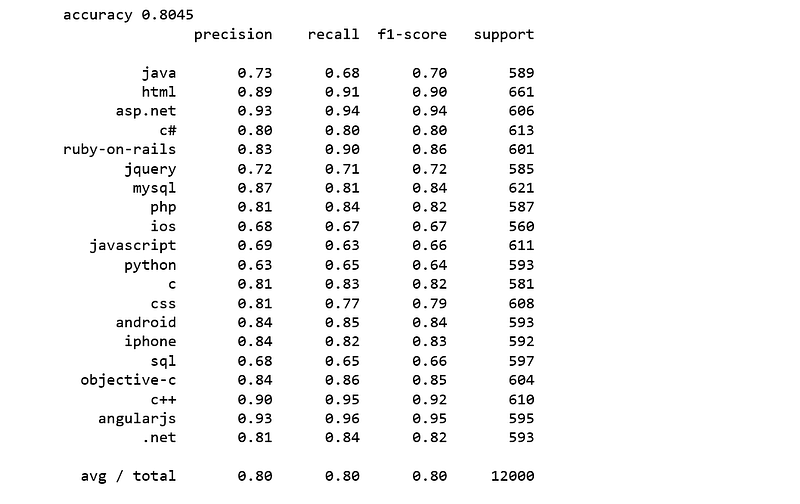Figure 12

We achieve an accuracy score of 80% which is 1% higher than SVM.

### BOW with Keras

Finally, we are going to do a text classification with Keras which is a Python Deep Learning library.

The following code were largely taken from a Google workshop. The process is like this:

• Separate the data into training and test sets.
• Use `tokenizer` methods to count the unique words in our vocabulary and assign each of those words to indices.
• Calling `fit_on_texts()` automatically creates a word index lookup of our vocabulary.
• We limit our vocabulary to the top words by passing a `num_words` param to the tokenizer.
• With our tokenizer, we can now use the `texts_to_matrix` method to create the training data that we’ll pass our model.
• We feed a one-hot vector to our model.
• After we transform our features and labels in a format Keras can read, we are ready to build our text classification model.
• When we build our model, all we need to do is tell Keras the shape of our input data, output data, and the type of each layer. keras will look after the rest.
• When training the model, we’ll call the `fit()` method, pass it our training data and labels, batch size and epochs.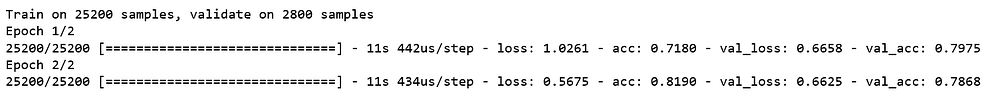Figure 13

The accuracy is:

```score = model.evaluate(x_test, y_test,
batch_size=batch_size, verbose=1)
print('Test accuracy:', score)```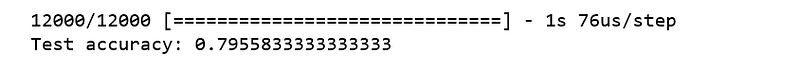Figure 14

So, which model is the best for this particular data set? I will leave it to you to decide.

Jupyter notebook can be found on Github. Have a productive day!

References:

Bio: Susan Li is changing the world, one article at a time. She is a Sr. Data Scientist, located in Toronto, Canada.

Original. Reposted with permission.

Related:

## Top Stories Past 30 DaysGet KDnuggets, a leading newsletter on AI, Data Science, and Machine Learning